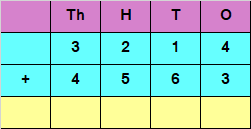a) ​7877​
b) ​8777
c) ​7777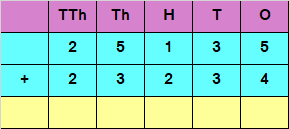a) 57389​
b) ​48369
c) 34783​

Q.3) Add the following : –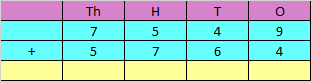a) ​13213
b) 12123​​
c) 13313​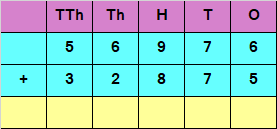a) 79651​
b) ​89691
c) ​89851

Q.5) ​The missing digit is ?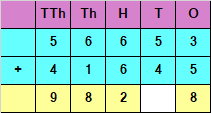a) 8
b) 9

Q.6) ​Find the estimated sum of 32 and 14?
a) 50
b) 40

Q.7) ​Find the estimated sum of 327 and 581?
a) 1000
b) 900

Q.8) ​Find the estimated sum of 2356 and 5272?
a) 7000
b) 6000

Q.9) Find the missing number.
___ + 0 = 84

Q.10) Find the missing number.
( ___ + 76 ) + 38 = 44 + ( 76 + 38 )

Adding the digits at ones place

4 + 3 = 7​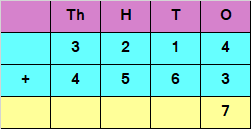​Adding the digits at tens place

1 + 6 = 7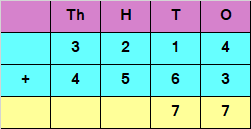​Adding the digits at hundreds place

2 + 5 = 7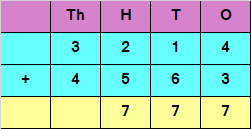​Adding the digits at thousands place

3 + 4 = 7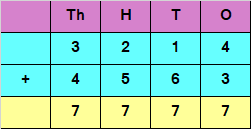​Hence, the sum of the given numbers is 7777

​Adding the digits at ones place

5 + 4 = 9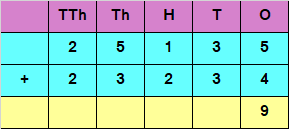​Adding the digits at tens place

3 + 3 = 6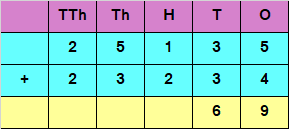​Adding the digits at hundreds place

1 + 2 = 3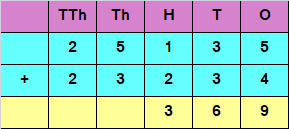​Adding the digits at thousands place

5 + 3 = 8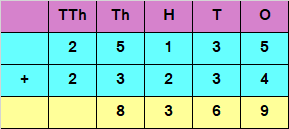​Adding the digits at ten thousands place

2 + 2 = 4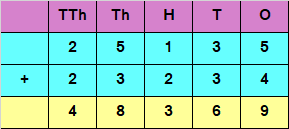​Hence, the sum of the given numbers is 48369

​Adding the digits at ones place ,
9 + 4 = 13
Since the number is more than 9, we  carry over 1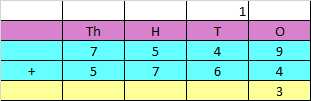Adding  the digits ​at tens place , and the carry over of 1
​4 + 6 + 1 = 11
Since the number is more than 9, we  carry over 1​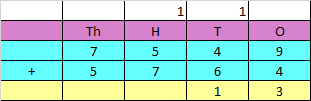​Adding the digits​ at hundreds place, and the carry over of 1​
5 + 7 + 1 = 13
Since the number is more than 9, we  carry over 1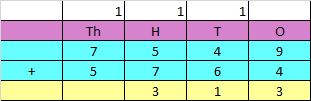​Adding  the digits​ at Thousands place, and the carry over of 1​
7 + 5 + 1 = 13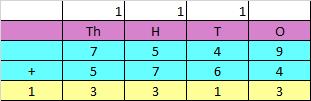​Hence, the sum of the given numbers  is 13313

Adding the digits at ones place
6 + 5 = 11
Since the number is more than 9, we carry over 1 to the tens place​​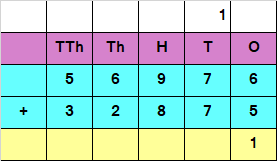​Adding the digits at tens place and the carry of 1
7 + 7 + 1 = 15
Since the number is more than 9, we carry over 1 to the hundreds place​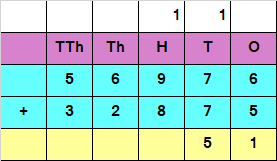​Adding the digits at hundreds place and the carry of 1
9 + 8 + 1 + 18
Since the number is more than 9, we carry over 1 to the thousands place​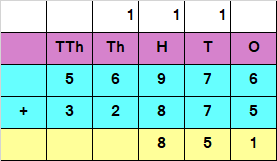​Adding the digits at thousands place and the carry of 1
6 + 2 + 1 = 9​Adding the digits at ten thousands place and the carry of 1
5 + 3 = 8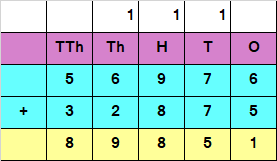​Hence, the sum of the given numbers is 89851

​Adding the digits at ones place
3 + 5 = 8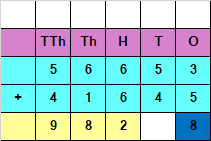​Adding the digits at tens place
5 + 4 = 9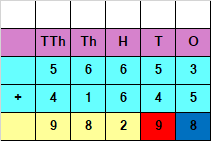​Hence, the missing digit is 9

​Step: 1 – Round off the given number to the nearest 10.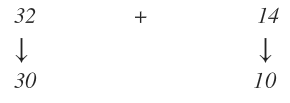Step: 2 – Add the numbers rounded off  at Step 1.

Estimated Sum = 30 + 10 = 40

​Step: 1 – Round off the given number to the nearest 100.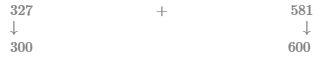Step: 2 – Add the numbers rounded off  at Step 1.

Estimated Sum = 300 + 600 = 900

Step: 1 – Round off the given number to the nearest 1000.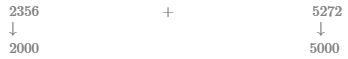Step: 2 – Add the numbers rounded off  at Step 1.

Estimated Sum = 2000 + 5000 = 7000

Given equation:
___ + 0 = 84
Based on the identity property of addition.
a + 0 = a
If we compare this with the given equation, we get
84 + 0 = 84